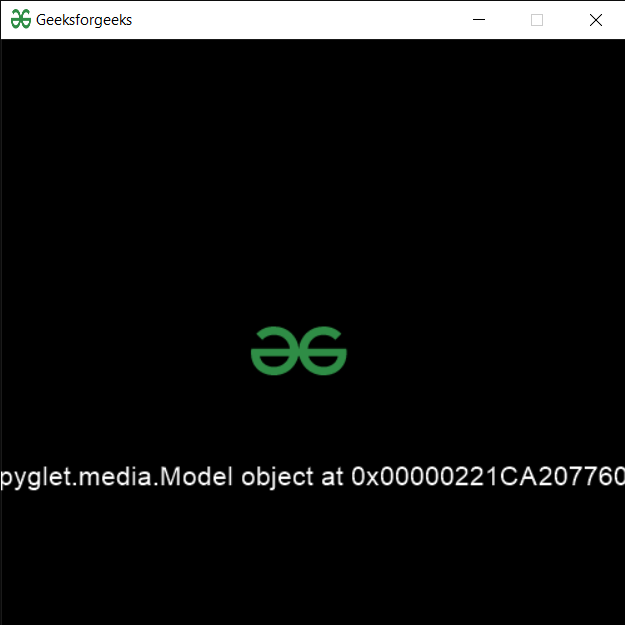• Last Updated : 06 Sep, 2021

In this article, we will see how we can load a 3-D model in PYGLET module in python. Pyglet is easy to use but powerful library for developing visually rich GUI applications like games, multimedia, etc. A window is a “heavyweight” object occupying operating system resources. Windows may appear as floating regions or can be set to fill an entire screen (fullscreen). In order to load a file i.e resource we use resource module of pyglet. This module allows applications to specify a search path for resources. Relative paths are taken to be relative to the application’s __main__ module. A 3D file format is used for storing information about 3D models. You may have heard of the most popular formats STL, OBJ, FBX, COLLADA, etc. They are widely used in 3D printing, video games, movies, architecture, academia, medicine, engineering, and earth sciences.

We can create a window object with the help of command given below

```# creating a window
window = pyglet.window.Window(width, height, title)```

In order to do this we use model method with the pyglet.resource
Syntax : resource.model(file_name)
Argument : It takes string i.e file name as argument
Return : It returns Model object

Below is the implementation

## Python3

 `# importing pyglet module``import` `pyglet``import` `pyglet.window.key as key``  ` `# width of window``width ``=` `500``  ` `# height of window``height ``=` `500``  ` `# caption i.e title of the window``title ``=` `"Geeksforgeeks"``  ` `# creating a window``window ``=` `pyglet.window.Window(width, height, title)``  ` `# text ``text ``=` `"Welcome to GeeksforGeeks"`` ` `# creating label with following properties``# font = cooper``# position = 250, 150``# anchor position = center``label ``=` `pyglet.text.Label(text,``                          ``font_name ``=``'Cooper'``,``                          ``font_size ``=` `16``,``                          ``x ``=` `250``, ``                          ``y ``=` `150``,``                          ``anchor_x ``=``'center'``, ``                          ``anchor_y ``=``'center'``)`  `# creating a batch``batch ``=` `pyglet.graphics.Batch()` `# loading geeksforgeeks image``image ``=` `pyglet.image.load(``'gfg.png'``)`  `# creating sprite object``# it is instance of an image displayed on-screen``sprite ``=` `pyglet.sprite.Sprite(image, x ``=` `200``, y ``=` `230``)``  ` `# on draw event``@window``.event``def` `on_draw():``      ` `    ``# clear the window``    ``window.clear()``      ` `    ``# draw the label``    ``label.draw()``    ` `    ``# draw the image on screen``    ``sprite.draw()``      ` `# key press event    ``@window``.event``def` `on_key_press(symbol, modifier):``  ` `    ``# key "C" get press``    ``if` `symbol ``=``=` `key.C:``        ` `        ``# printing the message``        ``print``(``"Key : C is pressed"``)``        ` `# image for icon``img ``=` `image ``=` `pyglet.resource.image(``"gfg.png"``)` `# setting image as icon``window.set_icon(img)` `# loading3-model file``value ``=` `pyglet.resource.model(``"model.stl"``)` `# setting text  of label``label.text ``=` `str``(value)` `# start running the application``pyglet.app.run()`

Output :My Personal Notes arrow_drop_up